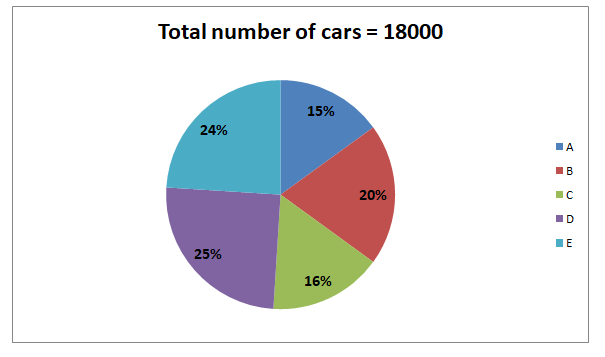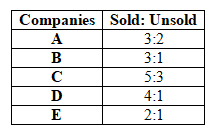# RRB PO Prelims Quantitative Aptitude Questions 2021 (Day-02)

Dear Aspirants, Our IBPS Guide team is providing new series of Quantitative Aptitude Questions for RRB PO Prelims 2021 so the aspirants can practice it on a daily basis. These questions are framed by our skilled experts after understanding your needs thoroughly. Aspirants can practice these new series questions daily to familiarize with the exact exam pattern and make your preparation effective.

Start Quiz

Wrong number series

Directions (01-05): Find out the wrong number in the following number series.

1) 2, 27, 224, 1575, 9452, 47285

A.27

B.47285

C.9452

D.224

E.1575

2) 36, 54, 132, 472.5, 2126.25, 11694.375

A.472.5

B.132

C.11694.375

D.2126.25

E.54

3) 11, 31, 151, 1049, 9422, 103643

A.151

B.31

C.103643

D.9422

E.1049

4) 342, 727, 1328, 2193, 3370, 4905

A.4905

B.727

C.2193

D.3370

E.1328

5) 456, 429, 365, 240, 20, -319

A.365

B.20

C.-319

D.456

E.429

Pie chart

Directions (06-10): Study the following information carefully and answer the questions given below.

The given pie chart shows the percentage of car manufactured in five different companies.The given table shows the ratio of the sold to unsold cars of five different companies.6) If the ratio of three different colors of cars Red, Blue and Pink sold in C and E is 3:2:1 and 3:4:2 respectively, then what is the difference between the number of blue cars sold in C and the number of Pink cars sold in E?

A.20

B.30

C.40

D.50

E.60

7) What is average number of cars sold in all the companies together?

A.2484

B.2498

C.2528

D.2520

E.2568

8) What is the ratio of the number of cars unsold in C and A together to the number of cars unsold in E and D together?

A.8:9

B.10:11

C.11:12

D.12:13

E.7:8

9) What is the difference between the number of unsold cars in B and D?

A.10

B.30

C.40

D.60

E.None of these

10) The number of sold cars in C is what percent of the total number of cars manufactured in all the companies together?

A.10%

B.12.56%

C.14%

D.18%

E.22.22%

2 * 9 + 9 = 27

27 * 8 + 8 = 224

224 * 7 + 7 = 1575

1575 * 6 + 6 = 9456

9456 * 5 + 5 = 47285

36 * 1.5 = 54

54 * 2.5 = 135

135 * 3.5 = 472.5

472.5 * 4.5 = 2126.25

2126.25 * 5.5 = 11694.375

11 * 3 – 2 = 31

31 * 5 – 4 = 151

151 * 7 – 8 = 1049

1049 * 9 – 16 = 9425

9425 * 11 – 32 = 103643

73 – 1 = 342

93 – 2 = 727

113 – 3 = 1328

133 – 4 = 2193

153 – 5 = 3370

173 – 6 = 4907

456 – 33 = 429

429 – 43 = 365

365 – 53 = 240

240 – 63 = 24

24 – 73 = -319

Directions (6-10) :

Number of car manufactured in A = 15/100 * 18000 = 2700

Number of sold car in A = 3/5 * 2700 = 1620

Number of unsold cars in A = 2/5 * 2700 = 1080

Number of car manufactured in B = 20/100 * 18000 = 3600

Number of sold car in B = 3/4 * 3600 = 2700

Number of unsold cars in B = 1/4 * 3600 = 900

Number of car manufactured in C = 16/100 * 18000 = 2880

Number of sold car in C = 5/8 * 2880 = 1800

Number of unsold cars in C = 3/8 * 2880 = 1080

Number of car manufactured in D = 25/100 * 18000 = 4500

Number of sold car in D = 4/5 * 4500 = 3600

Number of unsold cars in D = 1/5 * 4500 = 900

Number of car manufactured in E = 24/100 * 18000 = 4320

Number of sold car in E = 2/3 * 4320 = 2880

Number of unsold cars in E = 1/3 * 4320 = 1440

Number of blue cars sold in C = 2/6 * 1800 = 600

Number of pink cars sold in E = 2880 * 2/9 = 640

Difference = 640 – 600 = 40

Required average = (1620 + 2700 + 1800 + 3600 + 2880)/5

= 2520

Required ratio = (1080 + 1080):(1440 + 900)

= 2160:2340

= 12:13

Required difference = 900 – 900 = 0